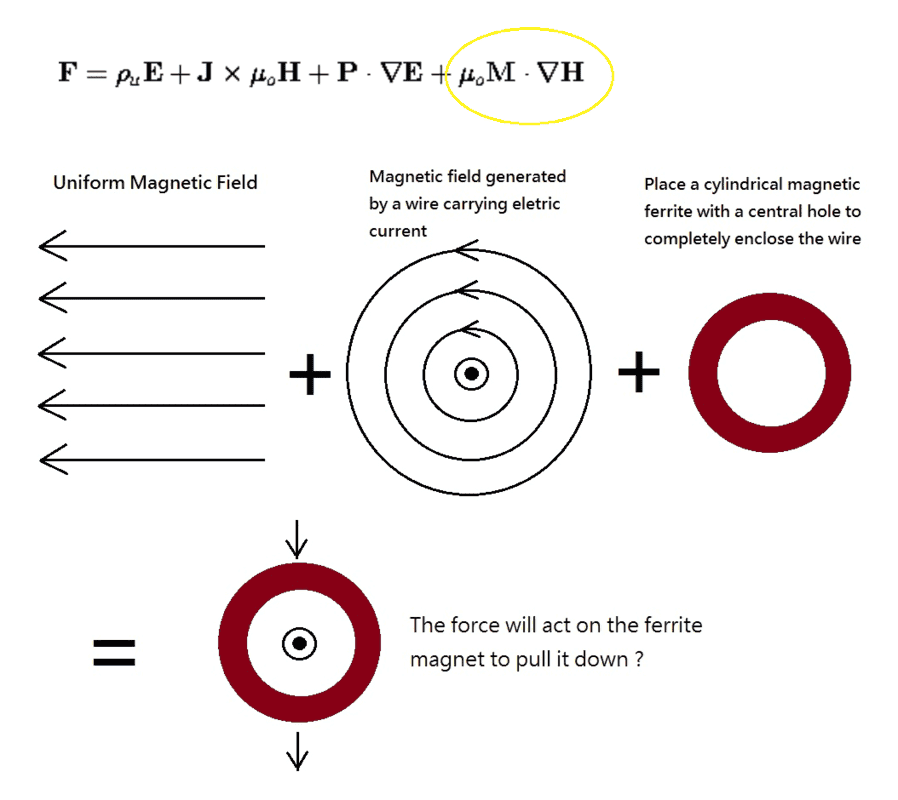# How Do You Calculate Forces when The Lorentz Force Doesn't Seem to Apply?

Dale
Mentor
2020 Award
Sometimes, in order to obtain accurate results, numerical solutions are inevitable.
I agree, this is a messy enough scenario that I suspect a numerical approach will be required. Also, @Keasy will need to use the macroscopic equations given in 11.10.19 here: http://web.mit.edu/6.013_book/www/book.html

•alan123hk and berkeman
I agree, this is a messy enough scenario that I suspect a numerical approach will be required. Also, @Keasy will need to use the macroscopic equations given in 11.10.19 here

Thank you for providing the link to the macroscopic equation in Section 11.10.19.

I think the equation can explain why the force acts on the ferrite magnet, as described in OP #1

Since an analytical solution may require a lot of mathematical work and simulation software, I am trying to infer abstract results based on the macroscopic equation by imagination.Last edited: